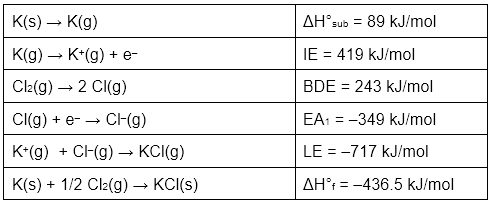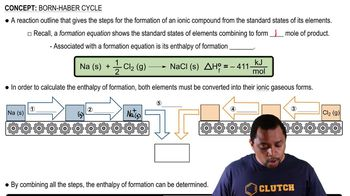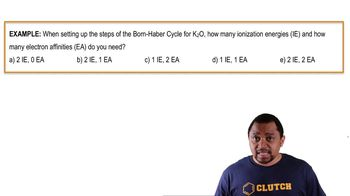Start typing, then use the up and down arrows to select an option from the list.
11:04 minutes
Problem 33
Textbook Question

# Given the following values for steps in the formation of CaO(s) from its elements, draw a Born–Haber cycle similar to that shown in Figure 6.7. Eea1 for O1g2 = -141 kJ/mol Eea2 for O1g2 = 745.1 kJ/mol Heat of sublimation for Ca1s2 = 178 kJ/mol Ei1 for Ca1g2 = 590 kJ/mol Ei1 for Ca1g2 = 1145 kJ/mol Bond dissociation energy for O21g2 = 498 kJ/mol Lattice energy for CaO1s2 = 3401 kJ/molVerified Solution
This video solution was recommended by our tutors as helpful for the problem above.
45views